# Reactive Power Cost Analysis

In this article, reactive power cost characteristics have been obtained developing the reactive power capability charts at different operating points and utilizing them to find lost opportunity cost... - Dr. Ashwani Kumar, Rajender KumarThe Independent System Operator (ISO) procures reactive power as an ancillary service from the synchronous generators and condensers to maintain security of the power system. Since cost is involved to procure reactive reserve services and to maintain the services, the service providers should be remunerated according to their reactive power supply to the system. For the recovery of the cost of reactive power, most of the utilities charge industrial consumers based on KVA demand and penalize the utilities for the poor power factor. This fixed cost recovery of reactive power is insufficient and in the competitive markets may not provide accurate price signal for the ancillary providers. Looking into the limitations of a reactive power price to be charged from the consumers based on power factor penalties, authors suggested the use of economic principles based on marginal theory.

However, the prices of reactive power based on the marginal price theory represent a small portion of the actual reactive power price. Reactive power has typical characteristics due to reactive power generation equipment and local characteristics of reactive power of loads and transmission system behavior; therefore, it is difficult to access its cost characteristics. For the assessment of reactive power cost, many authors proposed different techniques. A real time pricing method for reactive power using OPF method was proposd in.

The reactive power marginal price is typically less than 1% of the active power marginal price and depends strongly on the network constraints as proposed in. Gill et al. proposed a conceptual framework for remuneration and charging of reactive power cost. A cost based reactive power dispatch method is proposed for reactive power cost calculation and explicit and implicit cost of reactive support from generator and transmission system is also analyzed.

However the method of opportunity cost calculation of reactive support from generators is quite complex. A summary of different methods for reactive power pricing has been discussed using different objective functions for calculation of marginal prices of reactive power using optimal power flow based approach in.

A similar approach of generator cost model of reactive power support as discussed in is based on the opportunity cost of generator reactive power dispatch and is presented in. Capital cost remuneration of reactive compensators has also been incorporated in an objective function in.

Several methods for incorporating reactive power dispatch into nodal pricing and dispatch algorithms were proposed in. A method of cost-based reactive power pricing minimizing the cost of reactive power production of generators and capacitors are proposed in.

Ro has presented the reactive charging scheme composed of recovering capital cost and operational cost. The proper recovery of reactive power cost with help sustaining the ancillary service providers in the electricity markets. The cost must be decided based on the capability limits of the generators at each operating point due to the load and power factor variations. In this article, reactive power cost characteristics have been obtained developing the reactive power capability charts at different operating points and utilizing them to find lost opportunity cost.

The article presents: (i) Cost characteristics determination for synchronous generator based on capability charts, (ii) cost analysis of reactive power supported by synchronous condenser. The reactive power cost function has been obtained for reactive support of generator. These cost functions can be utilized for remuneration of reactive power supplied by the synchronous generators and condensers bidding in the ancillary service markets.

Reactive Power Capability of Synchronous Generators

Synchronous generators are the major sources of reactive power generation. The generators supply reactive power meeting the base load requirements and can be operated as synchronous condenser supplying reactive power to the system without any active power fed to the system. The reactive power supply of the generators are limited due to the armature current, field current limits, and end region heating limits. These limits need to be considered during the analysis of reactive power support and the cost of reactive power calculations.

Armature current limit

Armature current is responsible for heat loss in the windings as I2R losses and increases the temperature of the conductor. Therefore, the one of the limitations on generator rating is the maximum current that can be carried by the armature without exceeding the heating limitations. The real and reactive power supplied by the generator can be computed using the following expressions for complex power with the terminal voltage Et and armature current, It.The equations plotted on P-Q diagram are in the form of circle with centre on origin.

Field current limits

The field current is limited due to the heating of the windings. With terminal voltage Et and rated armature current Ia each equal to 1pu, the locus of the excitation current can be shown as a circle with centre on the Q axis as shown in Fig. 1. The figure also shows how the active power and reactive power capability curve is varying with the armature current and the field current. The reactive power generation for a cylindrical and salient pole generator is different and the real and reactive power generated, and the maximum reactive power can be computed for cylindrical pole and salient pole generator as follows:

1. a) For cylindrical pole rotor generator , the q-axis voltage, real, and reactive power is given by: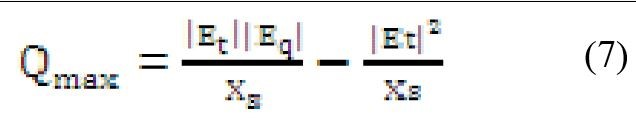For maximum reactive power generation limit, δ =0

1. b) Salient pole rotor synchronous generator or hydro generator: The induced voltage, the real and reactive power for salient pole generator can be obtained as: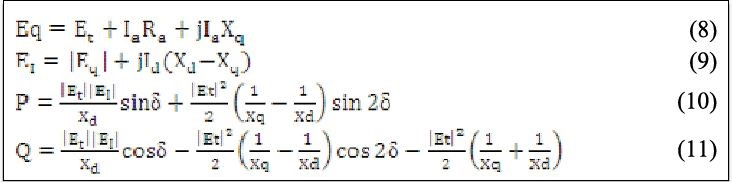For maximum reactive power generation limit δ =0Where,
Ifd = field current, P = active power pu, δ =load angle
Q = reactive power pu, Et=terminal voltage pu, Xs = synchronous reactance pu
Eq = no load generator voltage p.u., Xad = effective reactance (0.2 to 0.4 Xs)
Xq = reactance pu in q-axis, Xd = reactance pu in d-axis
For cylindrical rotor syn. Generator, the synchronous reactance Xs = Xd and nominal power factor is 0.9

End region heating limits

A third limit on the machine due to the localized heating in the end region of the armature imposes a limit on the operation of synchronous machine. The end turn leakage flux enters and leaves in the direction axial to the stator lamination. This cause’s eddy current to flow in the lamination and result in localized heating in the end region. In the over-excited condition, it keeps the retaining ring saturated, so that leakage flux is small. However, in the under excited region the field current is low and the retaining ring is not saturated, this causes increase in armature end leakage flux. The real and reactive power taking the end regions heating limits into account, the real and reactive power are obtained as follows:The end region heating limit is shown in green color in Fig. 1.

Synchronous condenser mode

A synchronous condenser is a synchronous machine running without a prime mover or mechanical load. In this situation, the machine only draws the real power to meet losses only. Practically, most of the machines are designed for one specific duty only, hence there are a finite limitations to the area within which any given machine may be operated successfully with about 20% to 30 % overload up to 30 minutes. When a machine is operated in the condenser mode, either in overexcited or under excited region by varying excitation current, it controls reactive power. Figure 2 shows that the condenser mode region is much smaller than the generator mode region. Region (i) and (iii) supply reactive power to the system in generator and condenser mode respectively. Region (ii) and (iv) absorb reactive power from the system in generator and condenser mode respectively. Negative P indicates that active power is absorbed from the grid to keep machine running in condenser mode and for supplying the losses.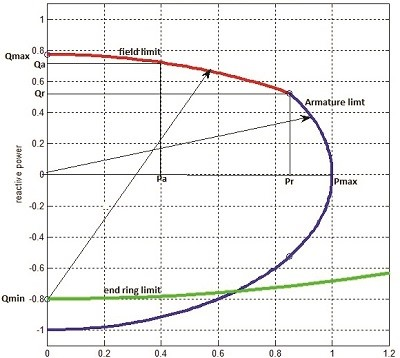Figure 1. Capability chart of the synchronous generator with three limits…

Cost model of synchronous generator reactive power supply

To provide the more reactive power, a generator may reduce the active power and may forego the cost of active power. This cost of forgo active power is known as the lost opportunity cost of generator. Since generator output is limited by its MVA rating, the accurate model of such opportunity cost should be derived from the generator capability curve at each operating point.

If a generator produces reactive power over and above its base case values, then its cost for generating reactive power should be equal to reduced active power (∇P) cost. The reactive power above the rated reactive power generation curve is limited by the field heating limit equation and below a rated reactive power generation, the curve is limited by armature limit equation. The lost opportunity cost can be determined above the rated reactive power requirements and below the maximum limit reactive power generation from the generators. Therefore, the reduction in active power has to be calculated by the field limit equation. From field current limit (4) and (5):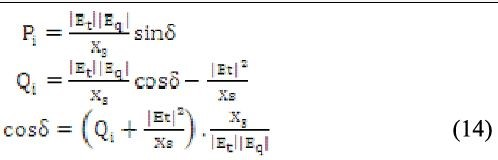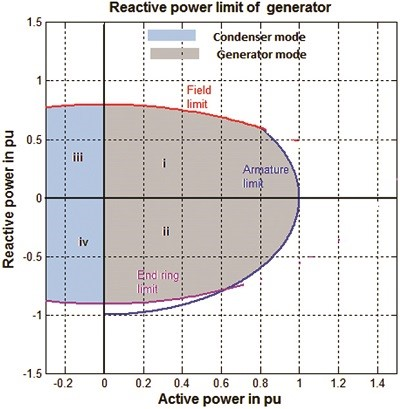Figure 2. Reactive power generation in generator and condenser mode…

The real power output limited by the field limits can thus be obtained as: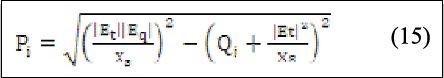Q will be maximum when Pi=0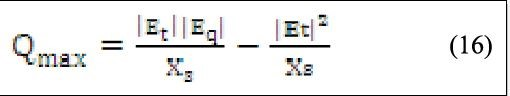Qrated =S*sin(Φ) (17)

The change in the real power can be obtained from its rated value to any operating value as:

∇P1 = Prated – P1 (18)

The cost of decrease in the real power output can be obtained using the fuel cost function.

Cost (∇P1) = a(∇P1)2 + b(∇P1) + C (19)

This cost of reduced active power shall be recovered from the reactive power support obtained from the generators as:The cost of reactive power following the capability chart of the generators can thus be plotted based on the reactive power support provided by the generators. Based on the data obtained, plot the graph Q vs Cost (Q) and by using of curve fitting toolbox in MATLAB, quadratic equation can be obtained for the reactive power cost as: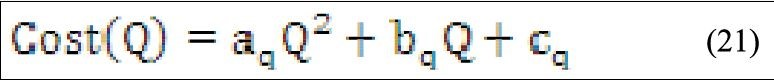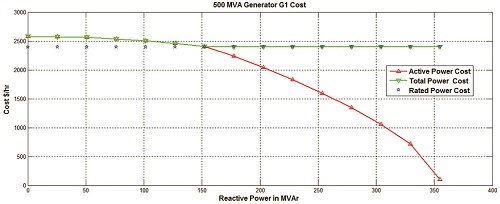Figure 3. Active power cost at any operating point and rated active power cost…Figure 4. 500 MVA Generator G1 Reactive power cost…Figure 5. Active power cost at any operating point and rated active power cost…

Cost characteristics curves for generators reactive support

The cost of real power and reactive power has been obtained for 500MVA and 60 MVA generators. Based on the capability chart of the 500 MVA generator, the real and the reactive power outputs have been obtained and the respective cost have been obtained at each operating point within the maximum reactive power limits of the generators. The cost of the active and the reactive power for the generators are obtained and are shown in Figs. 3 to Figs. 8. As observed from the figures, the lost opportunity cost can be recovered at every operating point on the capability curve of the generator. At maximum reactive power supply point, the real power given by the generator is zero.

The reactive power cost curve obtained for 500 MVA generators is shown in Fig. 4. Up to rated active power generation; the cost of reactive power is zero. Above the rated reactive power requirement, there is decrease in the real power generation and increase in the reactive power generation, thus the lost opportunity cost has to be recovered from the reactive power cost as an ancillary service. The cost of active power at rated values and at any other operating point on the capability curves of 60 MVA generators are also shown in Fig. 5 and 6. The reactive power cost curves obtained for 60 MVA generators are shown in Figs. 7 and 8. The cost of reactive power at rated active power generation is zero and increases with reduction of active power above the base case. Based on the results for both the 500MVA and 60 MVA generators, the cost characteristics for reactive power support have been obtained and the cost coefficients obtained for reactive power cost corves are given in Table 1.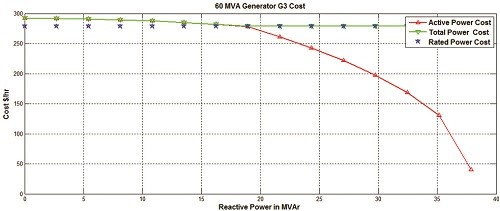Figure 6. Active power cost at any operating point and rated active power cost…Figure 7. 60 MVA Generator G2 Reactive power cost…Figure 8. 60 MVA Generator G3 Reactive power cost…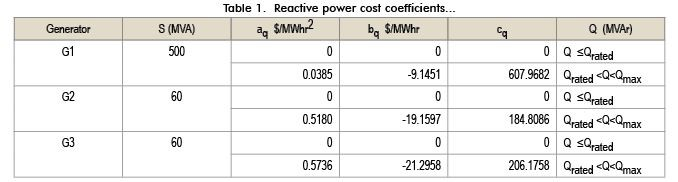Conclusions

In this article, reactive power cost functions have been obtained for the synchronous generators for their reactive support. The cost function for synchronous generators has been obtained based on their capability chart. The reactive support have been obtained for different sizes generators and using curve fit technique, quadratic cost functions have been obtained for synchronous generators. The cost of reactive power has also been obtained with generator in condenser mode. These cost functions can be utilized in the ancillary service markets to remunerate the generators for their reactive power support.